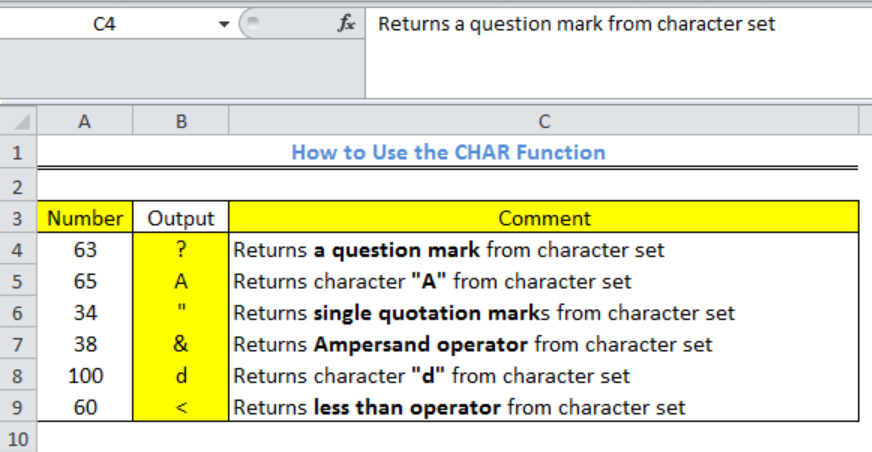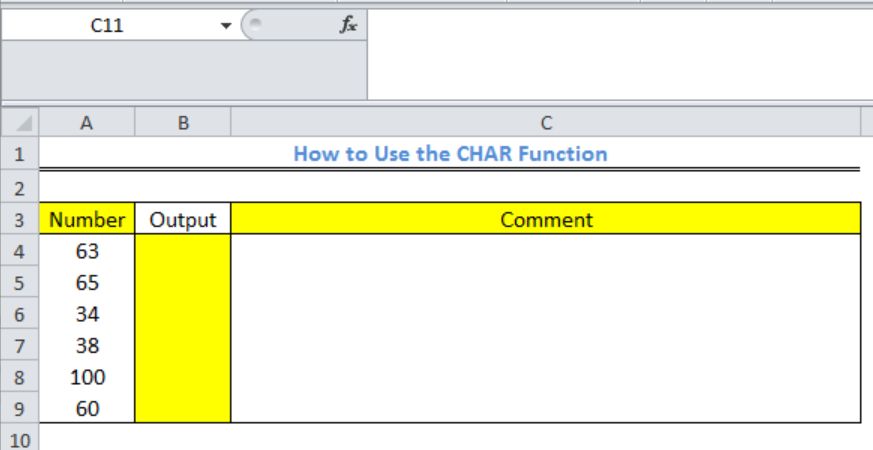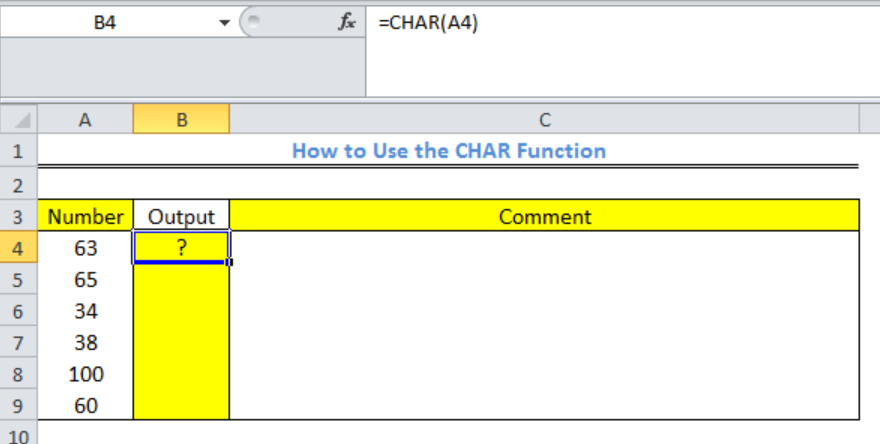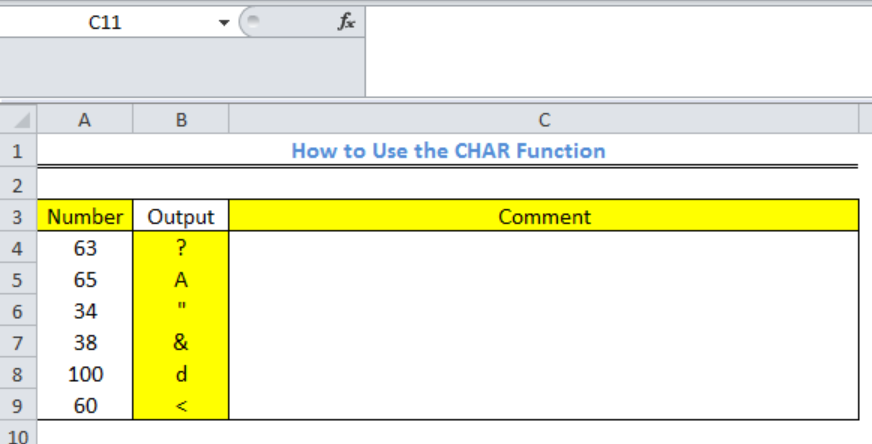Get instant live expert help with Excel or Google Sheets“My Excelchat expert helped me in less than 20 minutes, saving me what would have been 5 hours of work!”

#### Post your problem and you’ll get expert help in seconds.

Your message must be at least 40 characters
Our professional experts are available now. Your privacy is guaranteed.

# How to Use the Excel CHAR Function

We can use the excel CHAR function to return a character once we supply a valid character code. This is useful for specifying characters that are difficult to enter in a formula. This article will walk through the process of using the CHAR function.Figure 1: Result and Comment after using the Excel CHAR function

## Syntax

`=CHAR(number)`

## Formula

`=CHAR(A4)`

## Setting up the Data

The excel CHAR Function is valid for numbers between 1 and 255. Any number aside this range will produce a VALUE! error as the output. Use the UNICHAR function for numbers greater than 255.

• We will input the chosen numbers as shown in figure 2, Column A
• We will have the Output or result in Column B and Column C will be for comments based on the outputFigure 2: Setting up the Data for the Excel CHAR function

## Applying the Excel CHAR Function

• We will click on Cell A4
• We will input the formula below into Cell A4

`=CHAR(A4)`

• We will press the enter keyFigure 3: Result for Cell A4 Using the Excel CHAR function

• We will use the fill handle to drag down and copy the formula into the other output cellsFigure 4: Result of the Excel CHAR function

## Instant Connection to an Expert through our Excelchat Service

Most of the time, the problem you will need to solve will be more complex than a simple application of a formula or function. If you want to save hours of research and frustration, try our live Excelchat service! Our Excel Experts are available 24/7 to answer any Excel question you may have. We guarantee a connection within 30 seconds and a customized solution within 20 minutes.

### Did this post not answer your question? Get a solution from connecting with the expert.Another blog reader asked this question today on Excelchat:
Solution examplesI need a formula that has these 2 rules. If letter was rec'd between 1st and 15th = 1st of the month following letter rec'd date. If letter was rec'd between 16thst and end of the month= 1st of the 2nd month following letter rec'd date.
Solved by V. F. in 20 minsI need a column to total (Hours)(85) + (Travel)(.55) per line
Solved by F. Q. in 20 minsTrying to figure out what is wrong with my formula in Oct-ZD tab in column AY and BG.
Solved by O. Q. in 8 minsSo I have used vlookup to find the price of a product on a different sheet and then I have tried to times that by a quantity. e.g. =vlookup(d4,table35,2,false)*e4 This is the formula I typed in which worked for another list I did but for some reason it's not working now even though everything is practically the same, apart from the contents. Could you help?
Solved by B. U. in 60 minsI have a column containing date and time data together and i need to isolate the time data. When I tried to use text to column, it changed all the times to AM. There is an extremely large amount of data - over 550,000 rows so manually changing it won't work. The file is too large to attach.
Solved by S. E. in 40 mins## Subscribe to Excelchat.coAnother blog reader asked this question today on Excelchat: